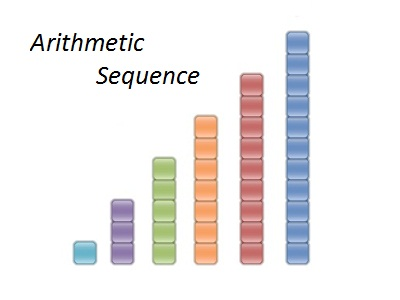# Arithmetic Sequence Calculator

#### Calculate Sum and nth term of Sequence

Use the free online Arithmetic Sequence Calculator tool to find nth term of sequence and the sum of the arithmetic sequence and n terms of progression.

## Definie Arithmetic Sequence

A sequence is a list of numbers in a particular order. Each number in a sequence is called a term. The first term is represented by a1, the second term is represented by a2 , and so on. an represents the nth term of the sequence. One kind of sequence is an arithmetic sequence. In an arithmetic sequence each term after the first is found by adding a constant, called the common difference, d, to the previous term.

A simple way to generate a sequence is to start with a number a, and add to it a fixed constant d, over and over again. This type of sequence is called an arithmetic sequence.

An arithmetic sequence is a sequence of the form: a, a + d, a + 2d, a + 3d, a + 4d, ........
The number a is the first term, and d is the common difference of the sequence.

## nth term of arithmetic sequence

The nth term of an arithmetic sequence is given by: an = a1 + (n – 1)d

The number d is called the common difference because any two consecutive terms of an arithmetic sequence differ by d, and it is found by subtracting any pair of terms an and an+1.
That is: d = an+1 – an

## Sum of arithmetic sequence

To find a formula for the sum, Sn, of the first n terms of an arithmetic sequence, we can write out the terms as
Sn = a + (a+d) + (a+2d) +......+[a+(n-1)d]

The final equation of Sum of arithmetic sequence is: Sn = n/2(a + an)

## Example for finding the terms of an Arithmetic Sequence

Example: Find the nth term, the fifth term, and the 100th term, of the arithmetic sequence determined by a = 2 and d = 3.

Solution: To find a specific term of an arithmetic sequence, we use the formula for finding the nth term. The nth term of an arithmetic sequence is given by an = a + (n – 1)d.

So, to find the nth term, substitute the given values a = 2 and d = 3 into the formula.

an = 2 + (n – 1)3

Now, to find the fifth term, substitute n = 5 into the equation for the nth term.

a5 = 2 + (5 – 1)3 = 14

Finally, find the 100th term in the same way as the fifth term.

a100 = 2 + (100 – 1)3 = 299### Spreading Knowledge Across the World

USA - United States of America  Canada  United Kingdom  Australia  New Zealand  South America  Brazil  Portugal  Netherland  South Africa  Ethiopia  Zambia  Singapore  Malaysia  India  China  UAE - Saudi Arabia  Qatar  Oman  Kuwait  Bahrain  Dubai  Israil  England  Scotland  Norway  Ireland  Denmark  France  Spain  Poland  and  many more....